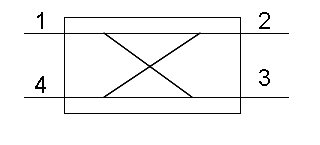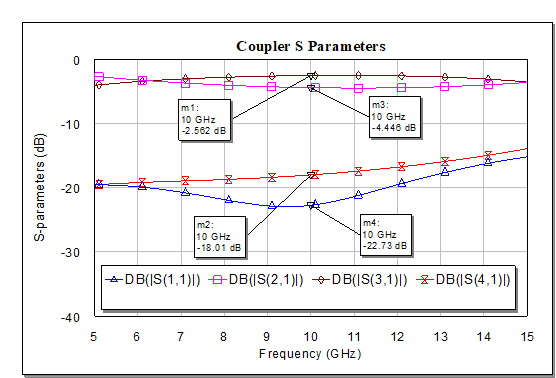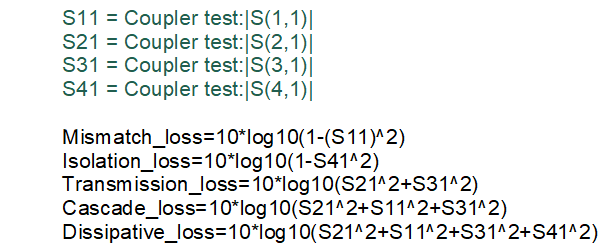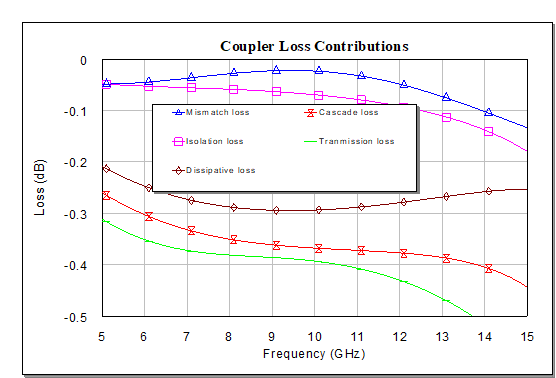# Coupler Loss

You are sitting at a design review and someone is presenting a 3 dB hybrid branchline coupler that has one port with -2.2 dB transmission, another with -4.4 dB, return loss of -16 dB and 21 dB isolation.

The boss asks, how much loss does this component have?  Someone responds "0.3dB", having averaged the two output ports and trying to correct for the 3dB power split. You pull up Excel on your laptop, type in the data and a formula you have committed to memory.  a few minutes later you ask to go back to the chart on the coupler, and state that the coupler simulation is actually lossless, and accuse the designer of using ideal components in his/her simulation.

"Whatdaya mean lossless", the designer says: "you can see that it does not provide 3dB power split, when you average the two, you get 3.3dB!"

First of all, you can't average terms that are expressed logarithmically.  If that were true, you could design coupler that provided 0 dB to one port and -6 dB to another port, which would clearly have gain.

Second, you need to account for the reflected power, and the power dumped out the isolation port when you compute coupler loss.

Let's look at the math for four port couplers, using the standard definition of ports: Port 1 is incident, Power 2 is the "through" port, Port 3 is the coupler port and Port 4 is the isolated port.The definitions below belong to Microwaves101, we have not seen this dealt with anywhere else.  In the expressions below, we are using magnitudes that are NOT in decebels.

Mismatch loss=(1-|S11|^2)

Isolation loss=1-|S41|^2

Dissipative loss=|S11|^2+|S21|^2+|S31|^2+|S41|^2

Transmission loss=|S21|^2+|S31|^2

Real loss is transmission loss, corrected for return loss, and is probably the most important quantity to focus on (unless your return loss is poor, say, worse than -16 dB).  In practice, when you cascade a coupler rwith something else, the return losses of components do not add up. If you are designing a system, you can tune out the return loss.

Now, let's put the terms into decibels:

Mismatch loss (dB)=10*log10(1-|S11|^2)

Isolation loss (dB)=10*log10(1-|S41|^2)

Dissipative loss dB=10*log10(|S11|^2+|S21|^2+|S31|^2+|S41|^2)

Transmission loss dB=10*log10(|S21|^2+|S31|^2)

## Coupler loss example

We had some S-parameters for an X-band Lange coupler laying around so we put them into a Microwave Office project.  Sorry, we can't show you the artwork, you'll have to design your own!  Below is a plot of its S-parameters, showing the design is over-coupled:Note that is you averaged S21 and S31 at 10 GHz, you might think the coupler has 0.5 dB loss, which is selling the design short.

Below, we entered the equations into Microwave Office, for a schematic that is called "Coupler test".And here, the loss components are plotted across frequency.  Note the isolation loss increasing with frequency, a direct result of the Lange coupler's issue with even and odd mode phase velocity dispersion. The loss for this coupler is less than 0.4 dB at 10 GHz if you go with "cascade loss".  That's probably closest to the truth.Author : Unknown Editor